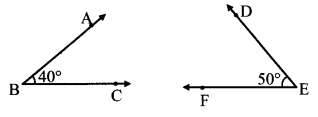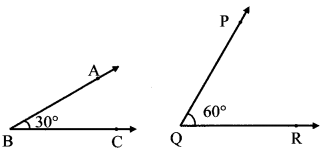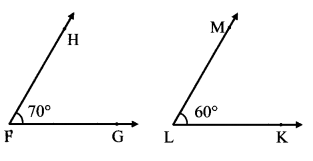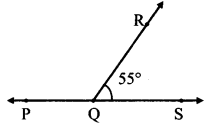# Maharashtra Board Practice Set 19 Class 7 Maths Solutions Chapter 4 Angles and Pairs of Angles

Balbharti Maharashtra State Board Class 7 Maths Solutions covers the 7th Std Maths Practice Set 19 Answers Solutions Chapter 4 Angles and Pairs of Angles.

## Angles and Pairs of Angles Class 7 Practice Set 19 Answers Solutions Chapter 4

Question 1.
Draw the pairs of angles as described below. If that is not possible, say why.
i. Complementary angles that are not adjacent.
ii. Angles in a linear pair which are not supplementary.
iii. Complementary angles that do not form a linear pair.
iv. Adjacent angles which are not in linear pair.
v. Angles which are neither complementary nor adjacent.
vi. Angles in a linear pair which are complementary.
Solution:
i.ii. Sum of angles in a linear pair is 180°.
i.e. they are supplementary .
∴ Angles in a linear pair which are not supplementary cannot be drawn.

iii.iv.v.vi. Angles in linear pair have their sum as 180° But, complementary angles have their sum as 90°.
∴ Angles in a linear pair which are complementary cannot be drawn.

Note: Problem No. i, iii, iv, and v have more than one answers students may draw angles other than the once given.

Maharashtra Board Class 7 Maths Chapter 4 Angles and Pairs of Angles Practice Set 19 Intext Questions and Activities

Question 1.
Observe the adjacent figure and answer the following questions: (Textbook pg. no. 29)1. Write the names of the angles in the figure alongside.
2. What type of a pair of angles is it?
3. Which arms of the angles are not the common arms?
4. m∠PQR = __.
5. m∠RQS = __.

Solution:

1. ∠PQR and ∠RQS
2. Angles in a linear pair
3. Ray QP and ray QS
4. 125
5. 55
Here, m∠PQR + m∠RQS = 125° + 55°
= 180°
∴The adjacent angles ∠PQR and ∠RQS are supplementary.